# What are neural networks and how do they work?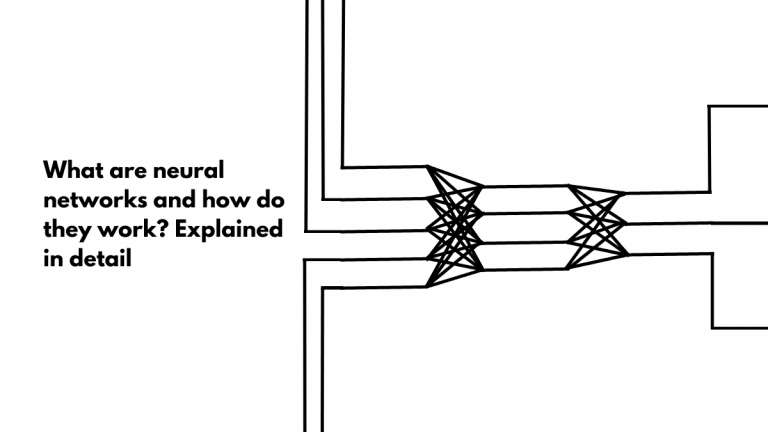Neural networks mimic how the neurons in a human brain works

In machine learning, an algorithm is only as good as the data it was trained on. Learning from experience is sorta the point of machine learning. Without ML, we would be manually programming a whole lot of “if” and “if-else” conditions for a computer to understand if an object is a car or a human being. And gradient descent is one of the most popular tools used in machine learning. But to understand gradient descent, let’s understand how artificial neural networks work.

# What are artificial neural networks or ANN?

As you may know, the human brain is made of billions of neurons. The connections between these neurons determine how our brain understands things and how it makes decisions. And artificial neural networks are an attempt to mimic the neural networks in our brain. And neural networks are the basis for everything from digital assistants to self-driving cars and just about everything in AI.

So to understand ANN, let’s have a look at how neural networks in our brain works.

The human brain is incredible plastic, it learns and changes from experience. While the total number of neurons in the brain remain more or less constant throughout our life, the connections between them keep changing. New connections may be formed, old connections may degrade or become stronger.

Each of these neurons has different parts with specific functions. But for our purpose, we can picture it as a line segment. The neuron gets signals from one end and sends out signals from the other. Now it doesn’t fit into our picture of a neuron as a line segment, but a single neuron can receive signals from and send signals to more than one neuron.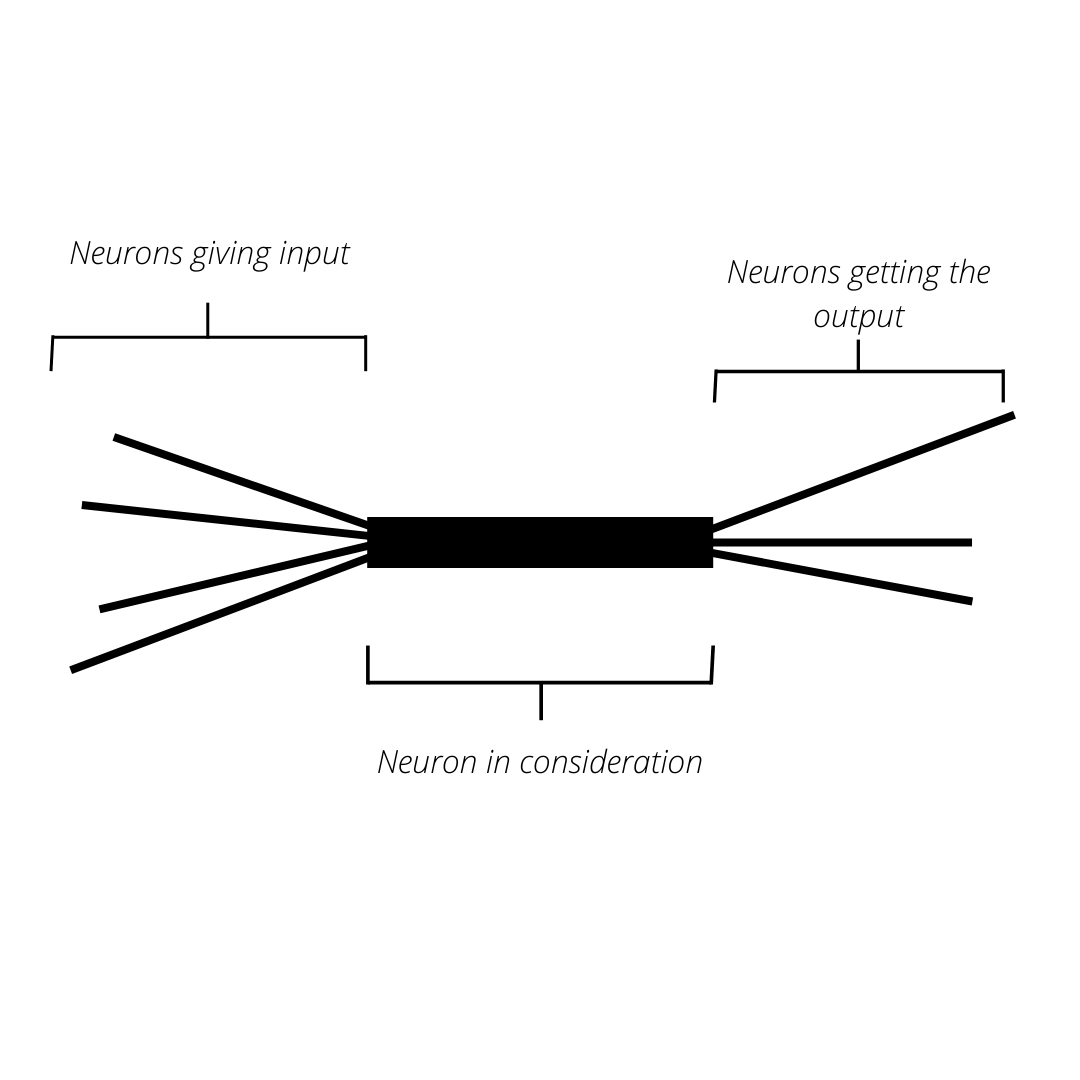A single neuron gets inputs from multiple neurons and gives outputs to multiple neurons

So as you can imagine, not all signals will be of equal magnitude. If multiple neurons simultaneously send signals, the magnitude will be higher. And neurons don’t all fire for every signal. They have a minimum magnitude of the signal that they need to get, called the action potential, for them to fire.

Now one of the thumb rules on how these connections are formed is “neurons that fire together, wire together”. Let’s have a look at the old example of Pavlov’s dog to understand this.

# Pavlov’s dog and training

The experiment is familiar. Ivan Pavlov measured the amount of saliva produced by his dog when offered food. Then for a while, before feeding the dog, he’d ring a bell.

After a while, he just rang the bell and measured the saliva production. The dog’s brain has come to associate the bell with food and started producing saliva when Pavlov rang the bell. Now to simplify things, we can picture a neuron that identifies food, another for producing saliva, and another for identifying the sound of the bell. (In reality, all of these would be complex neural networks themselves, and not individual neurons).

The neuron that identifies food and the one that produces saliva are already connected to each other. So when one fires, the other one fires too. But in this experiment, the neuron that identifies the sound of the bell was firing too. And it developed a connection with the food signalling neuron.

# How neural networks solve a problem

The brain solves all problems in a similar manner. For example, consider a situation when your brain is presented with a picture and is asked to identify if it is a dog. There are many factors that may indicate whether is a dog. For example, if it has 4 legs, it is likely that it’s a dog, but there are other animals with 4 legs too. Dogs usually have a coat of fur, but not always. A dog has two eyes, but most animals have two eyes. As you can see, these are all correlations, all of which combined together may help someone come to the conclusion that the picture is that of a dog’s.

All of these factors will have a neuron(once again, this is an oversimplification, in reality, each of these factors may be a neural network themselves), and they will all be connected to the neuron that finally says “yes this is a dog.” And the connections of all of these neurons to this final neuron will depend on how much the factors influence the decision.

For example, a thick coat of fur shows a higher correlation with a dog than four legs may, so the connection between the corresponding neurons will be stronger than the other pair of neurons. Now when all of the factors fire together, it will be enough to exceed the action potential of the final neuron, and the judgement is made.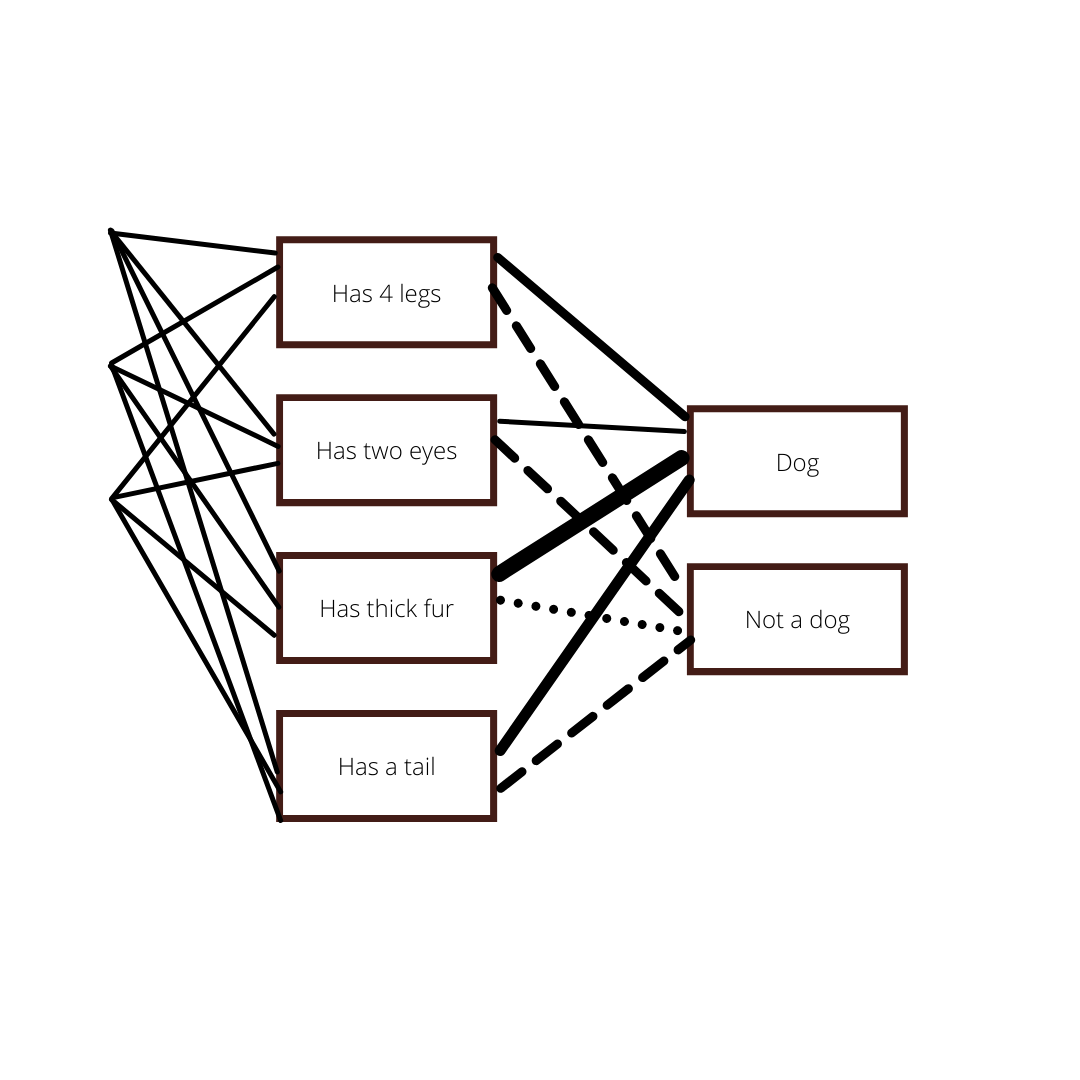A simplified depiction of neural network that determines if a picture is that of a dog or not

And as we discussed earlier, these connections are formed when they fire together. So when your brain is really young, it just forms these patterns, based on signals that come together at the same time. There may be some genetic predisposition towards certain kinds of patterns, but that is not very relevant when we try to understand artificial neural networks.

# How do artificial neural networks work?

The “tightness” or “strength” of connections between neurons in the brain is represented by, or rather corresponds to the weights in an artificial neural network. Let’s make it simpler.

Just like neurons, artificial neurons are also connected to many other neurons. A single neuron may get inputs from many neurons and may send its output to many others.

And depending on the importance of a factor in the decision, the signals get amplified with the weight associated with it. Consider the example of a neural network that determines if a picture is that of a dog. Two neurons, one representing that the figure has 4 legs and the other representing that the figure has a thick coat are connected to the neuron that lights up if it’s a dog. The decision making neurons are, of course, connected to input neurons.

Let’s say that the input neurons representing the four legs give an input, say 1, and the one representing the thick coat gives the same input. Now the input from the thick coat may get multiplied by, say 4, and the input from the four legs neuron may get multiplied by 2. (Representing that the presence of a thick coat increases the likelihood that the picture is that of a dog than the presence of 4 legs). So the total input now becomes 6.

# Threshold. Or bias

As with the natural neuron, the artificial neuron doesn’t fire every time it gets a signal. That is, taking the above example, the neuron that determines that the pic is indeed that of a dog won’t fire every time it gets a signal. The total signal it got was 6, now if the threshold for the neuron was 8, the neuron won’t fire (won’t give an output saying it’s a dog).

And when it comes to ANNs, the threshold is referred to as bias. (technically the negative of the threshold value is called the bias. I.e, if the threshold is 4, bias is -4)

So as you can see, three different components come together to decide if a neuron will fire or not, that is, the input from neurons connected to it, weights attached to these inputs, and the bias.

# Activation function of a neural network

The activation function is how the output from the neuron is calculated. Keep in mind that some aspects of this don’t correspond exactly to the natural neuron.

Generally, to calculate the activation function, you multiply the weights with the respective inputs and subtract the bias. If x1, x2, x3, … were the inputs, and w1, w2, w3 were the corresponding weights, and B was the bias, the activation function would be

y = x1w1 + x2w2 + x3w3……. — B

As you can imagine, the value of the function could range from negative infinity to positive infinity. But sometimes this would be restricted to other 1 or 0

Y = x1w1 + x2w2 + x3w3……. — B

y = 1, if Y>0

y = 0, if Y<0

There are other types of activation functions as well which may be used according to the situation.

# Training neural networks

A neural network is only as good as its training and the data used to train it. We know that in a natural neuron, the brain learns with experience; it forms new connections, and old connections grow stronger or weaker as it gains new information. How do we do that in an artificial neural network?

This is what we do with data. If you want to develop a neural network that can tell if there’s a dog in a picture, you’ll need a lot of pictures both with and without dogs in it. This will be the training data set. So as the input, we’ll feed these pictures to our network. But during training, we’ll also supply the expected output as well. For example, when we feed a picture with a dog as the input, the output would be “Detected dog.” Or in a more mathematical sense, 1 or 0.

Now training the network means finding out the exact combination of weights and biases associated with every neuron in the network so that the output worked. Of course, when you were feeding an image as input, it will be the pixel data that would be fed. So essentially, it would work like, you gave a number (or numbers) as input, and you would get another number as output. But with training data, you know both these numbers, which you’ll be using to find the weights.

Y = x1w1 + x2w2 + x3w3 + ….. B

Now if you know x1, x2, x3,…… and you knew Y, it’s like solving an equation. But since we have way too many variables, the process is complicated. Of course, we can try all the different combinations available for weights and biases, but that will take a lot of time and computing resources.

# But to identify the optimal solution, we have to define how perfect a solution is

That is, we have to know how incorrect the output is, or how far the actual output was from the expected output.

For example, let’s say we’re trying to develop an algorithm that predicts the salary of a person based on their age and experience. And for training, we have the data for 10000 people or so. For every single example in this data set, we’ll know the input and the output. To optimize the algorithm, we need to know the difference between the actual output, and the output.

This is the performance of a neural network and is defined by a cost function. The goal is to reduce this to a minimum for all the inputs by fine-tuning the weights and biases. The cost function shows how far the actual output is from the expected output for the entire set of training data. If it is for a single training example from the set, this is called the loss function.

# There are different types of cost functions that we can use in different situations.

For example, in the above situation, the output (the salary of an individual) could be just any number (more or less). Or let’s say we’re trying to estimate the distance between the camera and an object in the pic, or the actual size of a building from a picture. In all these situations, the output could be any number.

In such a situation, the loss function would be the difference between the actual output and the predicted output. (the difference between the actual salary and the predicted salary, or the actual distance and the predicted distance, or the actual size and the predicted size).

Loss function = actual output — predicted output

And the cost function may be the mean average of all of these errors, for all the data in the training data set. This is called Mean Error or ME. But as you can imagine, some of these errors may be negative. For example, in the above case, if for a training example the actual salary was 10k USD, but if the network predicted a salary of \$9k USD, the error is negative. And when negative errors come, the mean error may turn out to be zero.

In this situation, either the Mean Squared Error (MSE or the mean of square of all errors) or mean absolute error (mean of absolute errors for all training data) is taken.

# But what if the output is just 0 or 1? Or when the output cannot be any number?

This is the situation with classification problems. For example the situation we discussed earlier when a neural network has to judge the presence or absence of a dog in the pic? How do you determine the performance of a neural network, or how accurate the neural network is when the output is 0 or 1? Or if a neural network has to classify a set of pictures into that of a dog, a cat, and a fish?

In this situation, we use a different type of cost function. For a classification problem, the machine learning model will give the output as a probability distribution for a given input.

Let’s say, for instance, we give a picture as an input, the model will give an output saying, that its 23% likely to be a fish, 57% likely to be a dog, and 20% likely that its a cat (the one with the highest probability will be taken as the classification, that is dog, in this example).

We express this as

Y = [.23, .57, .20]

Now the expected output would be

Y’ = [0, 1, 0]

And this is how the output for a training example will look like for a picture of a dog.

For a picture of a fish, it will be

Y’ = [1, 0, 0]

And for a picture of a cat, it will be

Y’ = [0, 0, 1]

Now we have the expected output and the actual output.

Now we can calculate loss function as the distance between the two probability distributions.

Be prepared for a bit of matrix math here (it’s simple enough).

To find the loss function, we write the expected probability distribution as

[y1, y2, y3]

In the above example, it will be [0, 1, 0]

And we write the actual probability distribution after taking their logs

[logP1,

logP2,

logP3]

In the above training example it will be

[log .23,

log .57,

log .20]

To get the loss function, we multiply these two matrices.

[y1, y2, y3] * [logP1,

logP2,

logP3]

The loss = y1*logP1 + y2*logP2 + y3*logP3

Taking the above example, it will be

0*log.23 + 1*log.57 + 0*log.20

As you can imagine, with more classes, this equation will be longer.

The cost function would be the sum of loss functions for all training data.

Now we know if our neural network is accurate, and how inaccurate it is. The next step is to reduce this error and improve the performance of our neural network. And this is where we play around with the weights and biases to minimize the cost function.

Now as we discussed earlier, we can always try all the different combinations of weights and biases until we hit the right numbers. But as you can imagine this will take infinite time and computing resources. So we do this bit more systematically.

# This is where we use gradient descent.

Picture this scenario: you’re programming a rover to go to the deepest point in a pond, but you don’t know where the bottom of the pond is. The rover has sensors though, which can tell the angle it is facing, whether it is going down, or if it is level.

Picture three different axes for the pond. The coordinates of the rover according to these axes will keep changing as the rover moves, but at the very bottom, it will stop changing. That’s how the rover knows it is at the deepest point in the pond.

This is what we do with gradient descent. We don’t know what the values are, but we can see if the steps we take are taking us closer to it, and we know when we have found the values of these weights. Of course, we’ll have a lot more than 3 weights to figure out in most neural networks.

Now to go with the earlier example, the bottom of the pond is where the error is the minimum. And the steps we make into the pond are the changes we make to the weights. If we are moving in the right direction, the distance to the bottom, that is the error value, will go down. And we’ll know we’ve reached the minimum error once the error stops changing.

To apply gradient descent, we have to find the cost function. As we discussed earlier, we compute the cost using the training data. Keep in mind that we use the entire training data to obtain the cost function.

Let’s consider a simple example where we have to find just one unknown variable, a weight single neuron. Of course, in an actual neural network, there will be thousands of weights and biases to figure out.

Let’s say our cost function is

Now let’s picture this as a simple curve on a graph (the function here has a very specific curve, but picture it as just about any curve, maybe shaped like a 2D mountain)

Now, this graph may go up and down, (oversimplification), but for a given value of x, the function will have the lowest value. If you recall, this is a cost function or the error for the neural network, and x is the weight.

So let’s picture us standing on this graph. Let’s say we’re at x = 9. For this, the value of the function is 73. Of course, we can plot all the values of x and find out the lowest value of the function. But it’s easier if we know where the slope is towards, and move step by step in that direction (picture the earlier rover-going-down-into-the-pond analogy).

Now the first derivative at a point will give the slope of a function at that point. For the above function, it is

2x — 1

At x = 9, the slope of the above function is

17.

Keep in mind that the above graph is not that of the function we’re discussing, it's just a simple graph to show the slope and all.

Now based on this, we adjust the value of x.

So this is where we apply the formula for gradient descent

X1 = x0 — learning rate (gradient)

So x0 is the initial value of x, ie 9. And gradient is the slope of the function (17, at x = 9).

# Learning rate

The learning rate is the steps we take to reach the bottom of the pond. The learning rate plays a huge role in machine learning. Here’s how.

Let’s say that the rover takes small steps, checking after every step to see if it has reached the bottom. Every check takes a lot of time, so if the steps are small, it may take a very long time for the rover to reach the bottom.

But what if the steps are too big? Remember the pond is like a bowl(in this case, not so much, since there’s only one variable), so if the rover reaches the bottom and doesn’t stop, it will keep ongoing. Now it will have to travel back to reach the bottom. So yeah, the choice of learning rate matters.

# Back to the problem

So now we adjust the value of x with

X1 = 9 — (.1 * 17 )

Here we choose .1 as the learning rate

X1 = 7.3

Substituting in the cost function

= 46.99

As you can see, the error is low, but not zero.
So we repeat the process again

Slope =

2 * 7.3–1

13.6

X2 = X1 — .1 * 13.6

X2 = 7.3–1.36

= 5.94

Cost

= 30.3436

As you can see the error is going down.

Now there’s a question, why don’t we just wing it? Why bother doing all this calculation to see how much we should change the x, why don’t we take a guess?

Well, when it’s just one variable that might work. Of course, it’s hard to tell a computer to just “wing” it, but still. It can work.

# But what if we have more than one variable? What if we have to adjust more than one weight, as is the case with neural networks?

Consider a cost function

In this situation, the graph is a bit more complex. We can picture this as a 3-dimensional graph, where X and Y determine the Z values.

Or, we can picture this as a mountain range on a flat XY plane. Now we can divide this into the x and y-axis, and the altitude as the z-axis.

To determine the lowest value of Z, we have to use partial derivatives.

With partial derivative, we consider the slope only with respect to one axis and consider the other variable as a constant.

Now if you bring this back to the picture of mountains on top of an XY plane, and we keep the X-axis constant, we’ll essentially be taking a slice out of the mountain range, like how we cut a cake, except, no thickness. If we keep this slice of cake on its side on a piece of paper, now we have just one variable. And the slope is just like the slope for the function with a single variable, as we discussed above.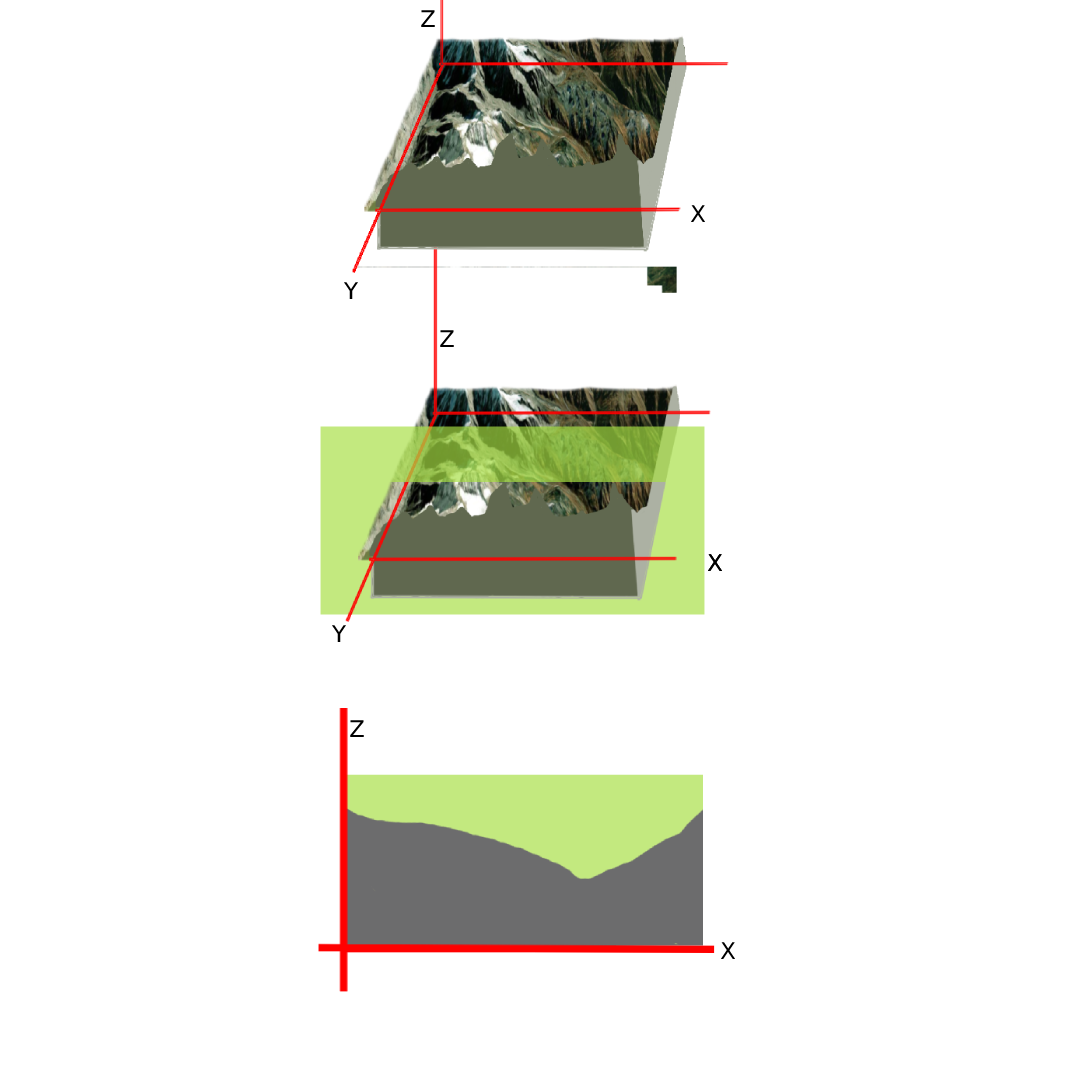Partial derivatives are just derivatives when you hold one variable as a constant.

# If you didn’t understand all of the above, no worries

It’s just since now we have two weights, we need two slopes to adjust these weights. And to find these slopes, you find the partial derivatives of the slope. And to find the partial derivative of the slope, you keep the other variable as a constant.

So if we take the earlier function f(x, y) = x 2+ y 2

Partial derivative with respect to x

d f(x,y)/dx = 2x

And partial derivative with respect to y

d f(x,y)/dy = 2y

Now let’s look at how we’re going to update the weights

We have two weights to update now

So for updating x

x1 = x0 + learning rate * partial derivative with respect to x

And for y

y1 = y0 + learning rate * partial derivative with respect to x

So let’s say that initially, we kept the weights as x = 5 and y = 10

So cost function will be

= 25 + 100 = 125

Updating x

X1 = 5 — (.1 * 10)

= 5–1

= 4

Updating y

Y1 = 10 — (.1 * 20)

= 10–2

= 8

Cost = 16 + 64

= 80

Similarly, the process will update the weights until the cost is very low

# How about when there are more weights in neural networks?

Well, as we discussed earlier, on average, neural networks may have more than 1000 weights. So how would you calculate them?

Exactly as we did with the two-weight situation.

We randomly assign weights

Calculate the cost function

Update the weights (same formula for the rest of the weights we well

Repeat until the cost is zero or close to zero

# Some words before you go

Now you know the importance of choosing the learning rate. Imagine all of these thousands of variables. And remember, the cost function would be a lot more complicated, the training data will have thousands of instances. So just finding the cost itself would take a lot of computing power. If you have to perform a lot of steps to find the lowest cost, that’s gonna complicate things. Therefore the choice of learning rate is very important while training neural networks.

Another factor is that what we discussed is just the simplest easiest function. If you consider the earlier example of a rover moving down into a pond or a mountain on an XY plane, they both will have the deepest point. But they may also have small pits where your rover may get stuck. It’s not the deepest point, but it’s deep compared to its immediate surroundings.

Something similar may happen with our mathematical operation as well. It may look like our cost function won’t go lower by much no matter how many more times we update the weights. And we may decide to just stop there. But it may not be the lowest value of the cost function. One of the things we can do to prevent this is to randomly choose the weights when you begin. There are other techniques as well which uses only a part of the training data at a time.

Originally published at https://blog.nuventure.in on December 29, 2021.

--

--

--

## More from Nuventure

Stay tuned to the latest news from the world of tech. Techie stories in layman’s terms, without confusing jargon(ok maybe some jargon).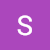## Steve

A tech writer with an unhealthy obsession towards smart gadgets and IoT

## Ordinary Least Squares and Normal Equations in Linear Regression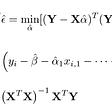## Confusion Matrix## Flipping pennies in Penney’s Game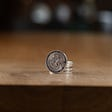## How To Create An MVP for Mental Health With Machine Learning